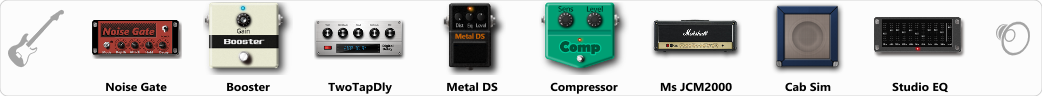# Mega Tone

Discussion in 'ToneLib-GFX presets' started by Mario Avila, Jun 3, 2019.

1. Mega Tone

GFX version: 3.0

Preset name: Mega Tone
Optimized for: Phones/Speaker

Effects chain:Trying to get a megadeth vibe

Effect: "Noise Gate" (Dynamics / Filter), active - "yes"
"Detect" = Gtr In
"Mode" = Auto
"Depth" = 50
"Threshold" = 50
"Attack" = 0
"Hold" = 5
"Decay" = 250

Effect: "Booster" (Dynamics / Filter), active - "yes"
"Gain" = 51

Effect: "TwoTapDly" (Delay), active - "yes"
"Time" = Note sync, 1/16
"Feedback" = 49
"Tone" = 81
"Sens" = 50
"TapTime" = 1.9
"Mix" = 14

Effect: "Metal DS" (Overdrive / Distortion), active - "yes"
"Dist" = 0
"Bass" = 66
"Middle" = 70
"Treble" = 62
"Level" = 50

Effect: "Compressor" (Dynamics / Filter), active - "yes"
"Sense" = 22
"Level" = 50

Effect: "Ms JCM2000" (Amp simulators), active - "yes"
"Gain" = 50
"Bass" = 70
"Middle" = 38
"Treble" = 56
"Presence" = 65
"Master" = 50
"Output" = 50
"Level (dB)" = 0

Effect: "Cab Sim" (Cabinets), active - "yes"
"Model" = 1x12" US Delux
"Level (dB)" = 5

Effect: "Studio EQ" (Dynamics / Filter), active - "yes"
"31 Hz" = -15
"62 Hz" = -2
"125 Hz" = 2
"250 Hz" = 5
"500 Hz" = -5
"1 kHz" = -3
"2 kHz" = 4
"4 kHz" = -4
"8 kHz" = 0
"16 kHz" = 0
"above 16 kHz" = 0
"Level (dB)" = 0

Note: This is a ToneLib-GFX preset file, you will need to download and install the ToneLib-GFX software to use the preset.

#### Attached Files:

• ###### Mega_Tone.tlgfx
File size:
705 bytes
Views:
7,471
2. hey man thats a good sound..

Mario Avila likes this.
3. Thanks for trying it out dude.

4. Sounding cool!!!